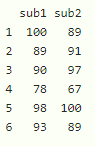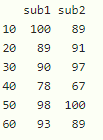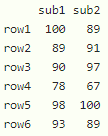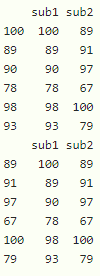# Substitute DataFrame Row Names by Values in Vector in R

• Last Updated : 30 Sep, 2021

In this article, we will discuss how to substitute dataframe row names by values in a vector in R programming language.

Dataframe in use:We can substitute row names by using rownames() function

Syntax:

rownames(dataframe) <- vector

where,

• dataframe is the input dataframe
• vector is the new row values

Example: R program to substitute the rownames of the dataframe

## R

 `# create a dataframe with 6 rows and 2 columns``data = ``data.frame``(sub1=``c``(100, 89, 90, 78, 98, 93),``                  ``sub2=``c``(89, 91, 97, 67, 100, 89))` `# consider the vector``vec = ``c``(10, 20, 30, 40, 50, 60)` `# substitute the row names by values in a vector``rownames``(data) = vec` `# display dataframe``print``(data)`

Output:Example: R program to substitute the rownames of the dataframe

## R

 `# create a dataframe with 6 rows and 2 columns``data = ``data.frame``(sub1=``c``(100, 89, 90, 78, 98, 93),``                  ``sub2=``c``(89, 91, 97, 67, 100, 89))` `# consider the vector``vec = ``c``(``"row1"``, ``"row2"``, ``"row3"``, ``"row4"``, ``"row5"``, ``"row6"``)` `# substitute the row names by values in a vector``rownames``(data) = vec` `# display dataframe``print``(data)`

Output:We can also replace the row names by values in a dataframe

Syntax:

rownames(dataframe) <- dataframe\$column_name

where

• dataframe is the input dataframe
• column_name is the column of the dataframe

Example: R program to substitute rownames of the dataframe using column

## R

 `# create a dataframe with 6 rows and 2 columns``data = ``data.frame``(sub1=``c``(100, 89, 90, 78, 98, 93),``                  ``sub2=``c``(89, 91, 97, 67, 100, 79))` `# substitute the row names by sub1 column``rownames``(data) = data\$sub1` `# display dataframe``print``(data)` `# substitute the row names by sub2 column``rownames``(data) = data\$sub2` `# display dataframe``print``(data)`

Output:My Personal Notes arrow_drop_up